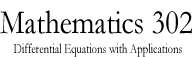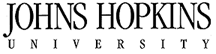Math 302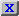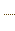HW3.pdfStatement on DisabilitiesStatement on EthicsHomework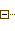Java ToolsAn example of a circuitAn example of a circuitAn example of a circuitAn Application to Population DynamicsAn application to Population DynamicsSlope Field CalculatorODE 2D CalculatorODE 2D CalculatorODE 3D CalculatorODE 3D CalculatorSlope Field CalculatorSlope Field CalculatorSolution VerifierSolution Verifier 2DSolution Verifier 2DSolution VerifierA Lotke-Volterra SystemA Lotke-Volterra systemLabor Managed Oligopoly - Two firmsODE 3D Calculator2D Map CalculatorA model of sunamiA model of sunamiThe three body problemThe two body problemThe two body problemVan der Pol EquationVan der Pol EquationList of parameters of the JOde AppletBrowser testGenerated Documentation (Untitled)ODE 2D CalculatorUsing Marek Rychliks's Applet for Euler's MethodJOde Manual PageJOde - An Applet for Studying Ordinary Differential Equations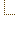ODE 2D CalculatorSyllabus
 Math Dept. Home Help room

# Slope Field Calculator - Framed Version

Hopefully, you see the Applet running in a frame outside of your browser. You must remain on this page until your done using the applet, or the changes you make will be lost.

View Instructions on using the Applet

Switch to unframed version

Marek Rychlik (rychlik@u.arizona.edu)